# Building a Content-Based Book Recommendation Engine

In this blog, we will see how we can build a simple content-based recommender system using Goodreads data.

By Dhilip Subramanian, Data Scientist and AI Enthusiast

If we plan to buy any new product, we normally ask our friends, research the product features, compare the product with similar products, read the product reviews on the internet and then we make our decision. How convenient if all this process was taken care of automatically and recommend the product efficiently? A recommendation engine or recommender system is the answer to this question.

Content-based filtering and collaborative-based filtering are the two popular recommendation systems. In this blog, we will see how we can build a simple content-based recommender system using Goodreads.com data.

### Content-based recommendation system

Content-based recommendation systems recommend items to a user by using the similarity of items. This recommender system recommends products or items based on their description or features. It identifies the similarity between the products based on their descriptions. It also considers the user's previous history in order to recommend a similar product.

Example: If a user likes the novel “Tell Me Your Dreams” by Sidney Sheldon, then the recommender system recommends the user to read other Sidney Sheldon novels, or it recommends a novel with the genre “non-fiction”. (Sidney Sheldon novels belong to the non-fiction genre).

As I mentioned above, we are using goodreads.com data and don’t have user reading history. Hence, we have used a simple content-based recommendation system. We are going to build two recommendation systems by using a book title and book description.

We need to find similar books to a given book and then recommend those similar books to the user. How do we find whether the given book is similar or dissimilar? A similarity measure was used to find this.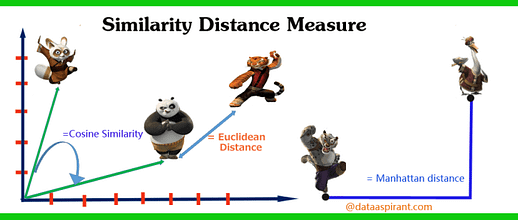Source: dataaspirant

There are different similarity measures are available. Cosine Similarity was used in our recommender system to recommend the books. For more details on the similarity measure, please refer to this article.

### Data

I scraped book details from goodreads.com pertaining to business, non-fiction and cooking genres.

``````# Importing necessary libraries
import pandas as pd
import numpy as np
import pandas as pd
import numpy as np
from nltk.corpus import stopwords
from sklearn.metrics.pairwise import linear_kernel
from sklearn.feature_extraction.text import CountVectorizer
from sklearn.feature_extraction.text import TfidfVectorizer
from nltk.tokenize import RegexpTokenizer
import re
import string
import random
from PIL import Image
import requests
from io import BytesIO
import matplotlib.pyplot as plt
%matplotlib inline

#Checking the shape of the file
df.shape()``````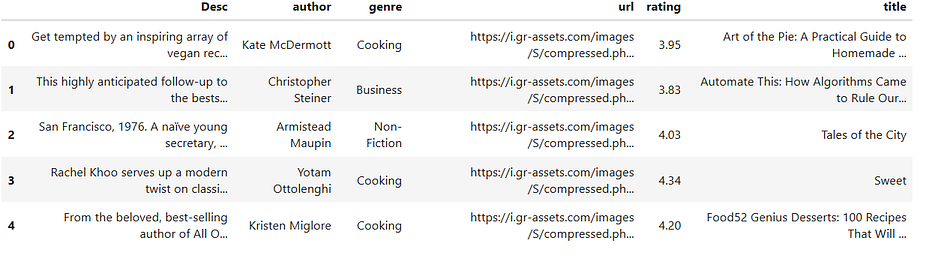Total 3592 books details available in our dataset. It has six columns:

• title -> Book Name
• Rating -> Book rating given by the user
• Genre -> Category(Type of book). I have taken only three genres like business, non-fiction and cooking for this problem
• Author -> Book Author
• Desc -> Book description
• url -> Book cover image link

### Genre distribution

``````# Genre distribution
df['genre'].value_counts().plot(x = 'genre', y ='count', kind = 'bar', figsize = (10,5)  )``````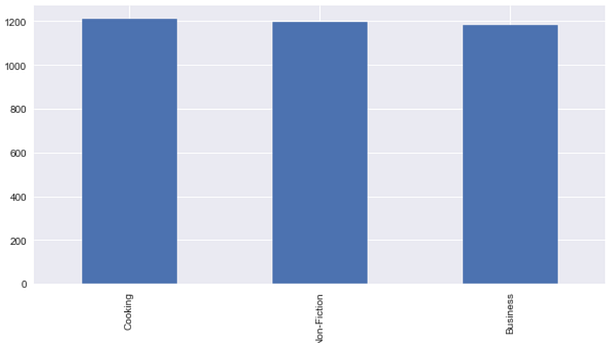Printing the book title and description randomly

``````# Printing the book title and description randomly
df['title'] 
df['Desc']``````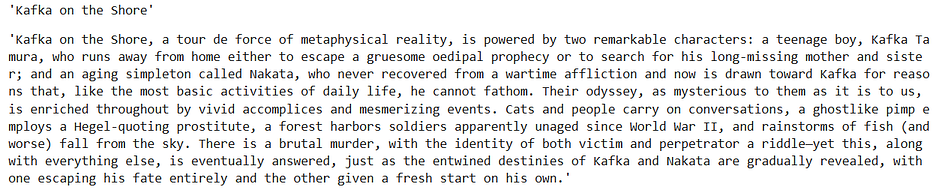``````# Printing the book title and description randomly
df['title'] 
df['Desc']``````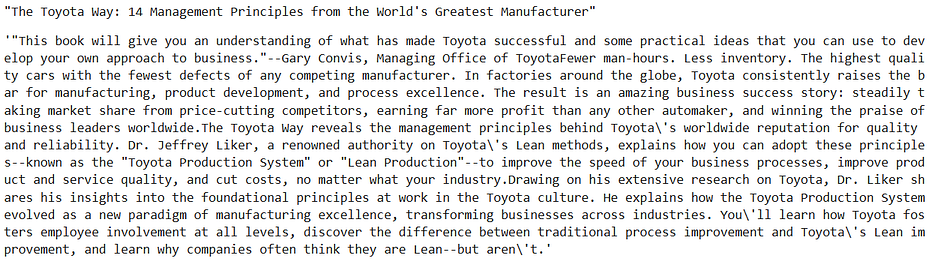### Book description — Word count distribution

``````# Calculating the word count for book description
df['word_count'] = df2['Desc'].apply(lambda x: len(str(x).split()))# Plotting the word count
df['word_count'].plot(
kind='hist',
bins = 50,
figsize = (12,8),title='Word Count Distribution for book descriptions')``````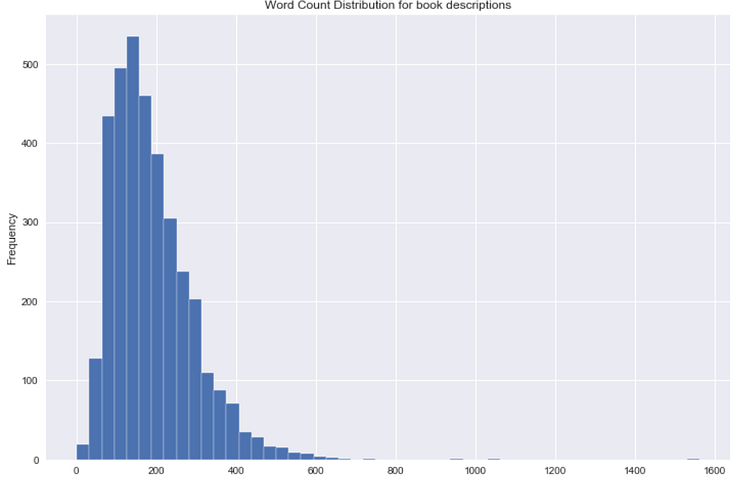We don’t have many lengthy book description. It is clear that goodreads.com provides short descriptions.

### The distribution of top part-of-speech tags in the book descriptions

``````from textblob import TextBlob
blob = TextBlob(str(df['Desc']))
pos_df = pd.DataFrame(blob.tags, columns = ['word' , 'pos'])
pos_df = pos_df.pos.value_counts()[:20]
pos_df.plot(kind = 'bar', figsize=(10, 8), title = "Top 20 Part-of-speech tagging for comments")``````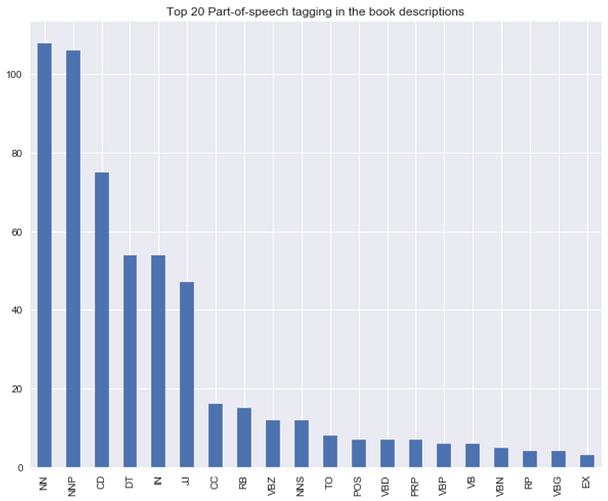### Bigram distribution for the book description

``````#Converting text descriptions into vectors using TF-IDF using Bigram
tf = TfidfVectorizer(ngram_range=(2, 2), stop_words='english', lowercase = False)
tfidf_matrix = tf.fit_transform(df['Desc'])
total_words = tfidf_matrix.sum(axis=0)
#Finding the word frequency
freq = [(word, total_words[0, idx]) for word, idx in tf.vocabulary_.items()]
freq =sorted(freq, key = lambda x: x, reverse=True)
#converting into dataframe
bigram = pd.DataFrame(freq)
bigram.rename(columns = {0:'bigram', 1: 'count'}, inplace = True)
#Taking first 20 records

#Plotting the bigram distribution
bigram.plot(x ='bigram', y='count', kind = 'bar', title = "Bigram disribution for the top 20 words in the book description", figsize = (15,7), )``````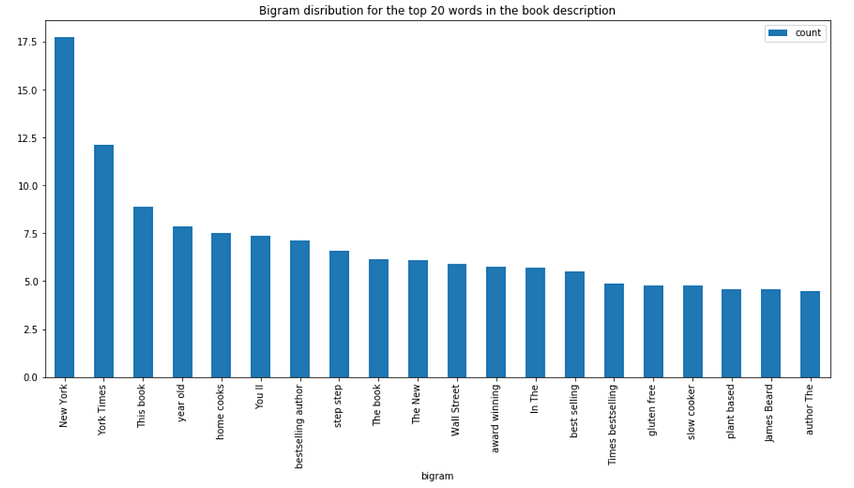### Trigram distribution for the book description

``````#Converting text descriptions into vectors using TF-IDF using Trigram
tf = TfidfVectorizer(ngram_range=(3, 3), stop_words='english', lowercase = False)
tfidf_matrix = tf.fit_transform(df['Desc'])
total_words = tfidf_matrix.sum(axis=0)
#Finding the word frequency
freq = [(word, total_words[0, idx]) for word, idx in tf.vocabulary_.items()]
freq =sorted(freq, key = lambda x: x, reverse=True)#converting into dataframe
trigram = pd.DataFrame(freq)
trigram.rename(columns = {0:'trigram', 1: 'count'}, inplace = True)
#Taking first 20 records

#Plotting the trigramn distribution
trigram.plot(x ='trigram', y='count', kind = 'bar', title = "Bigram disribution for the top 20 words in the book description", figsize = (15,7), )``````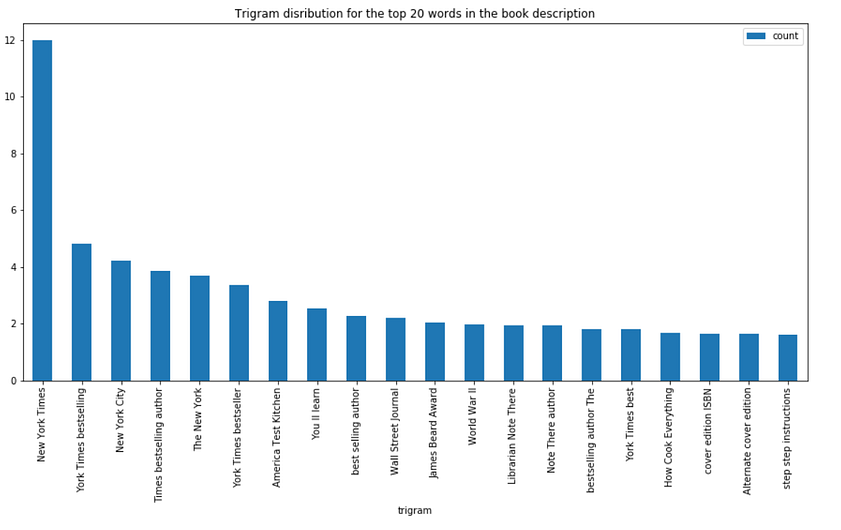### Text Preprocessing

Cleaning the book descriptions.

``````# Function for removing NonAscii characters
def _removeNonAscii(s):
return "".join(i for i in s if  ord(i)<128)

# Function for converting into lower case
def make_lower_case(text):
return text.lower()

# Function for removing stop words
def remove_stop_words(text):
text = text.split()
stops = set(stopwords.words("english"))
text = [w for w in text if not w in stops]
text = " ".join(text)
return text

# Function for removing punctuation
def remove_punctuation(text):
tokenizer = RegexpTokenizer(r'\w+')
text = tokenizer.tokenize(text)
text = " ".join(text)
return text

# Function for removing the html tags
def remove_html(text):
html_pattern = re.compile('<.*?>')
return html_pattern.sub(r'', text)

# Applying all the functions in description and storing as a cleaned_desc
df['cleaned_desc'] = df['Desc'].apply(_removeNonAscii)
df['cleaned_desc'] = df.cleaned_desc.apply(func = make_lower_case)
df['cleaned_desc'] = df.cleaned_desc.apply(func = remove_stop_words)
df['cleaned_desc'] = df.cleaned_desc.apply(func=remove_punctuation)
df['cleaned_desc'] = df.cleaned_desc.apply(func=remove_html)``````

### Recommendation engine

We are going to build two recommendation engines using the book titles and descriptions.

1. Convert each book title and description into vectors using TF-IDF and bigram. See here for more details on TF-IDF
2. We are building two recommendation engines, one with a book title and another one with a book description. The model recommends a similar book based on title and description.
3. Calculate the similarity between all the books using cosine similarity.
4. Define a function that takes the book title and genre as input and returns the top five similar recommended books based on the title and description.

### Recommendation based on book title

``````# Function for recommending books based on Book title. It takes book title and genre as an input.def recommend(title, genre):

# Matching the genre with the dataset and reset the index
data = df2.loc[df2['genre'] == genre]
data.reset_index(level = 0, inplace = True)

# Convert the index into series
indices = pd.Series(data.index, index = data['title'])

#Converting the book title into vectors and used bigram
tf = TfidfVectorizer(analyzer='word', ngram_range=(2, 2), min_df = 1, stop_words='english')
tfidf_matrix = tf.fit_transform(data['title'])

# Calculating the similarity measures based on Cosine Similarity
sg = cosine_similarity(tfidf_matrix, tfidf_matrix)

# Get the index corresponding to original_title

idx = indices[title]# Get the pairwsie similarity scores
sig = list(enumerate(sg[idx]))# Sort the books
sig = sorted(sig, key=lambda x: x, reverse=True)# Scores of the 5 most similar books
sig = sig[1:6]# Book indicies
movie_indices = [i for i in sig]

# Top 5 book recommendation
rec = data[['title', 'url']].iloc[movie_indices]

# It reads the top 5 recommended book urls and print the images

for i in rec['url']:
response = requests.get(i)
img = Image.open(BytesIO(response.content))
plt.figure()
print(plt.imshow(img))``````

Let’s recommend based on the book title “Steve Jobs” and genre “Business”:

``recommend("Steve Jobs", "Business")``

Output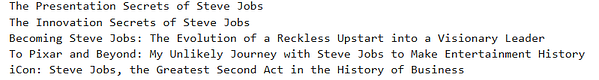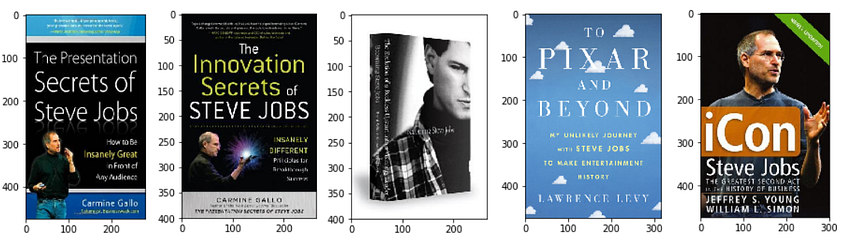We have given the book "Steve Jobs" as input and the model recommends other Steve Jobs books based on the similarity existing in the book title.

### Recommendation based on book description

We are using the same above function by converting book description into vectors.

``````# Function for recommending books based on Book title. It takes book title and genre as an input.def recommend(title, genre):

global rec
# Matching the genre with the dataset and reset the index
data = df2.loc[df2['genre'] == genre]
data.reset_index(level = 0, inplace = True)

# Convert the index into series
indices = pd.Series(data.index, index = data['title'])

#Converting the book description into vectors and used bigram
tf = TfidfVectorizer(analyzer='word', ngram_range=(2, 2), min_df = 1, stop_words='english')
tfidf_matrix = tf.fit_transform(data['cleaned_desc'])

# Calculating the similarity measures based on Cosine Similarity
sg = cosine_similarity(tfidf_matrix, tfidf_matrix)

# Get the index corresponding to original_title

idx = indices[title]# Get the pairwsie similarity scores
sig = list(enumerate(sg[idx]))# Sort the books
sig = sorted(sig, key=lambda x: x, reverse=True)# Scores of the 5 most similar books
sig = sig[1:6]# Book indicies
movie_indices = [i for i in sig]

# Top 5 book recommendation
rec = data[['title', 'url']].iloc[movie_indices]

# It reads the top 5 recommend book url and print the images

for i in rec['url']:
response = requests.get(i)
img = Image.open(BytesIO(response.content))
plt.figure()
print(plt.imshow(img))``````

Let’s recommend based on the book “Harry Potter and the Prisoner of Azkaban” and the genre “Non-Fiction”:

``recommend("Harry Potter and the Prisoner of Azkaban", "Non-Fiction")``

Output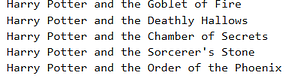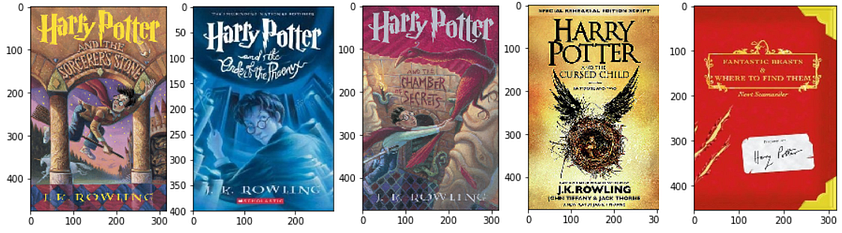We provided “Harry Potter and the Prisoner of Azkaban” and “Non-Fiction” as inputs, and the model recommends five other Harry Potter books which are similar to our input.

Let’s get one more recommendation:

``recommend("Norwegian Wood", "Non-Fiction")``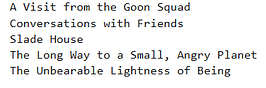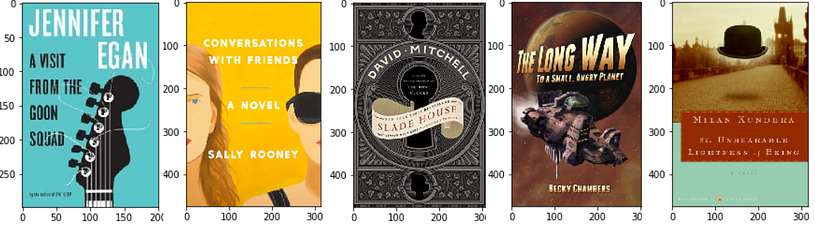The above model recommends five books similar to Norwegian Wood based on the description.

This is just a simple basic level recommender system. Real-world recommendation systems are more robust and advanced. We can further improve the above by adding other metadata like author and genre.

Also, we can do text-based semantic recommendation using Word2Vec. I have used Word2Vec and built a recommendation engine. Please check here.

Bio: Dhilip Subramanian is a Mechanical Engineer and has completed his Master's in Analytics. He has 9 years of experience with specialization in various domains related to data including IT, marketing, banking, power, and manufacturing. He is passionate about NLP and machine learning. He is a contributor to the SAS community and loves to write technical articles on various aspects of data science on the Medium platform.

Original. Reposted with permission.

Related: